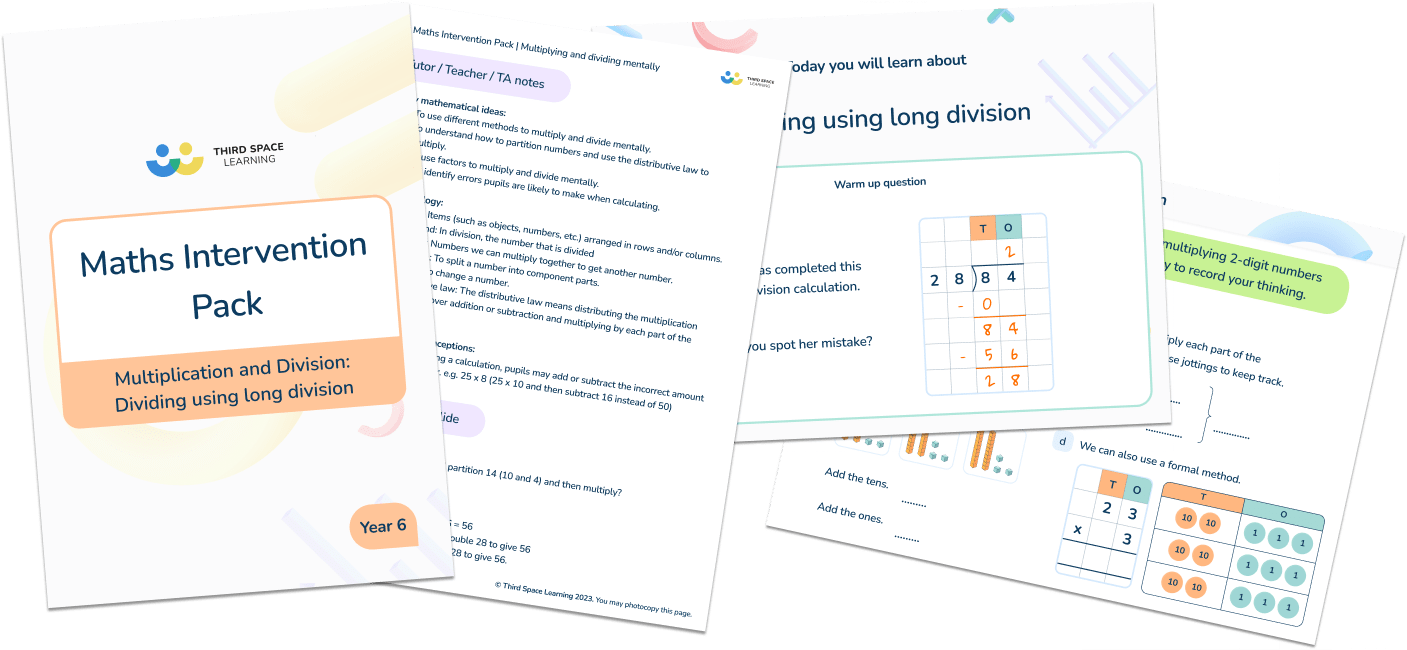# Maths Intervention Pack Multiplication and Division

### Years 2 to 6• Lessons include:
• Using 2x, 5x and 10x table (Year 2)
• Multiplying 2-digit numbers (Year 3)
• Using pictures to solve problems (Year 3)
• Multiplying and dividing mentally (Year 4)
• Using multiplication and division facts up to 12 x 12 (Year 4)
• Dividing using short division with exchange (Year 5)
• Multiplying 2-digit numbers using long multiplication (Year 5)
• Dividing using long division (Year 6)
• Using mental strategies to divide (Year 6)
• Each lesson contains teaching notes, answers and slides to work through with your pupils.
• Lessons follow exactly the same format that the Third Space Learning specialist maths tutors use in their one to one online maths tutoring.